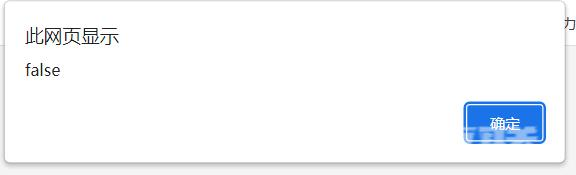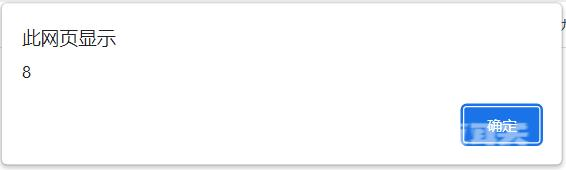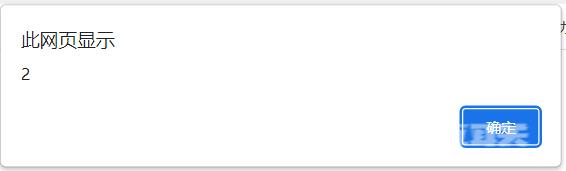﻿ JavaScript中常见的高阶函数总结 - 自由资讯

# JavaScript中常见的高阶函数总结

• 前言
• map函数
• reduce函数
• filter函数
• sort
• every方法
• find方法
• findIndex方法
• foreach方法
• 总结

## 前言

```function add(x, y, f) {
return f(x) + f(y);
}
//x = -5;
//y = 6;
//f = Math.abs;
//f(x) + f(y) ==> Math.abs(-5) + Math.abs(6) ==> 11;
//return 11;

//用代码验证一下：

## map函数

map()方法定义在JavaScript的数组中，我们调用Array的map()方法，传入我们自己的函数，就得到了一个新的数组。

``` function pow(x) {
return x * x;
}

var arr = [1, 2, 3, 4, 5, 6, 7, 8, 9];
```## reduce函数

```let arr=[6,8,8,6,9,6]
let a=arr.reduce(function(x,y){
return x+y;
})
```## filter函数

filter函数可以说是数组的一个过滤函数，它会将接收的函数作用于每一个元素，然后根据返回的布尔值决定这个元素留下还是丢掉。

filter()接收的回调函数，其实可以有多个参数 ，通常我们仅使用 第一个参数 表示元素的位置和数组本身。

```var arr = [1,2,1,2,1,2,1,2];
var r = arr.filter(function (x) {
return x % 2 == 0;
});
```## sort

sort主要用于排序

```//从小到大排列
let arr=[1,3,1,2,3,6,4,3]
let arr1=arr.sort((a,b)=>a-b)
``````//从大到小排列
let arr=[1,3,1,2,3,6,4,3]
let arr1=arr.sort((a,b)=>b-a)
```## every方法

```let arr=[4,6,8,8]
let result=arr.every(function(a){
return a>6
})
```## find方法

```let arr=[4,6,8,9]
let result=arr.find(function(a){
return a>6
})
```## findIndex方法

``` let arr=[4,6,8,9]
let result=arr.findIndex(function(a){
return a>6
})
```## foreach方法

forEach() 方法对数组的每个元素执行一次给定的函数

```const items = ['item1', 'item2', 'item3'];
const copy = [];
//传统方式
for (let i=0; i<items.length; i++) {
copy.push(items[i]);
}
// foreach方法
items.forEach(function(item){
copy.push(item);
});
```

﻿